Next: Deducing Self-Obscured SURFACEs Up: Feature Visibility Analysis Previous: Feature Visibility Analysis

## Deducing Back-Facing SURFACEs

Deducing whether planar SURFACEs are back-facing or not is simple: if the predicted surface normal points away from the camera, then the SURFACE is not visible.

 Let: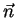be the model SURFACE normal ((0, 0, -1) by definition)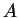be the coordinate transformation from the SURFACE's local system to that of the whole object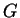be the transformation from the object's local system to that of the camera= a nominal point on the SURFACE in local coordinates Then: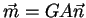is the predicted normal orientationis the view vector from the camera to the point on the SURFACE Test: if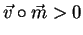, then the SURFACE is back-facing

For curved SURFACEs, we test the normal at each point on the boundary. By the segmentation assumptions (Chapter 3), the surface varies smoothly within the boundaries, so if all points on the boundary and the nominal central point are back-facing, then the interior of the surface almost always is as well.

A problem occurs with the combination of nearly tangential SURFACEs and parameter misestimation. Here, SURFACEs predicted as visible may not always be so, and vice versa. This case can be detected, by detecting surface normals oriented nearly perpendicular to the line of sight at the surface boundary. If a SURFACE is determined to be tangential, hypothesis construction does not require image evidence for it.

Classifying the visibility of curved SURFACEs follows this logic: If a substantial portion of a curved SURFACE is front-facing, then call it "front-facing". If it is not "front-facing" and a substantial portion of the SURFACE is tangential, then call it "tangential". Otherwise, call it "back-facing". The ideal form of this test is:

 Let: T = set of points whose surface normals are nearly perpendicular to the three dimensional line of sight (i.e. the tangential points) F = set of points whose surface normals face the viewer, but are not in T (i.e. the front-facing points) B = set of points whose surface normals face away from the viewer, but are not in T (i.e. the back-facing points) Then: If empty(F) and empty(T), then back-facing (i.e. never seen) If empty(F) and not(empty(T)), then tangential (i.e. possibly seen) If not(empty(F)), then front-facing (i.e. always seen)

Because of parameter estimation errors, some compromises in the above ideal algorithm are made:

• thresholds are added to decide the visibility class of each vector
• thresholds are added to decide the visibility class of the whole SURFACE

The algorithm to classify individual vectors is:

 Let: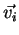be the line of sight to point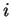be the predicted surface normal vector at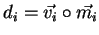Then: if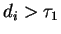, then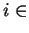B (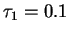) if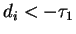, thenFT otherwise

 The classification of the whole SURFACE is obtained by: Let:= size(B)= size(F)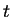= size(T)Then: if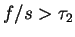, then front-facing (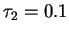) else if, then tangential (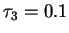) else back-facing

When this classification was applied to the objects with their estimated reference frames in the test image, surface visibility was correctly deduced. The results are shown in Table 9.11.

Table 9.11: Predicted SURFACE Visibility
Object SURFACE Visibility
robbody front-facing = {robbodyside(1)}
tangential = {robbodyside(2)} *1
robshldbd front-facing = {robshldend,robshould2}
tangential = {robshould1} *1
robshldsobj front-facing = {robshoulds(1)}
tangential = {robshoulds(2)} *1
upperarm front-facing = {uside(2),uends,uedgel(1)}
back-facing = {uside(1),uendb,uedgel(2)}
tangential = {uedges(1),uedges(2)}
lowerarm front-facing = {lsideb,ledgea,lendb}
back-facing = {lsidea,ledgeb}
trashcan front-facing = {tcanoutf(1),tcaninf(1),
tcanbot(1)}
back-facing = {tcanbot(2)}
tangential = {tcanoutf(2),tcaninf(2)} *1
*1 - largely back-facing curved SURFACE has tangential sidesNext: Deducing Self-Obscured SURFACEs Up: Feature Visibility Analysis Previous: Feature Visibility Analysis
Bob Fisher 2004-02-26